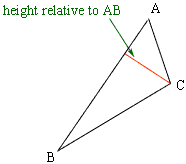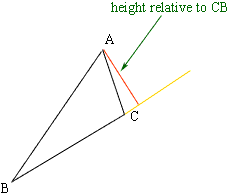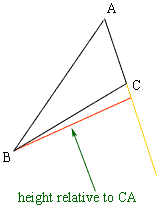Quandaries and Queries what is the definition of height of a triangle? Hi, The height of a triangle depends on which side you select as the base. If you have a triangle, select two of the vertices and call the line segment joining these vertices the base. The (perpendicular) distance from the third vertex of the triangle to the line containing the first two vertices is called the height. You should really call it the height relative to the base you have choosen.Base ABBase BCBase CA Penny Go to Math Central